# RS Aggarwal Solutions for Class 6 Chapter 10 Ratio, Proportion and Unitary Method Exercise 10B

The second exercise of Chapter 10 helps us understand the concept of proportion. We know that if two ratios are equal, they are in proportion and the symbol which is used is ‘: :’ or ‘=’. With the help of the solutions prepared by faculty at BYJU’S, the students can score well in the Class 6 exam. RS Aggarwal solutions help in improving self-study methods among students which helps to maintain better academic scores. Students can access RS Aggarwal Solutions for Class 6 Chapter 10 Ratio, Proportion and Unitary Method Exercise 10B PDF for free.

## Download PDF of RS Aggarwal Solutions for Class 6 Chapter 10 Ratio, Proportion and Unitary Method Exercise 10B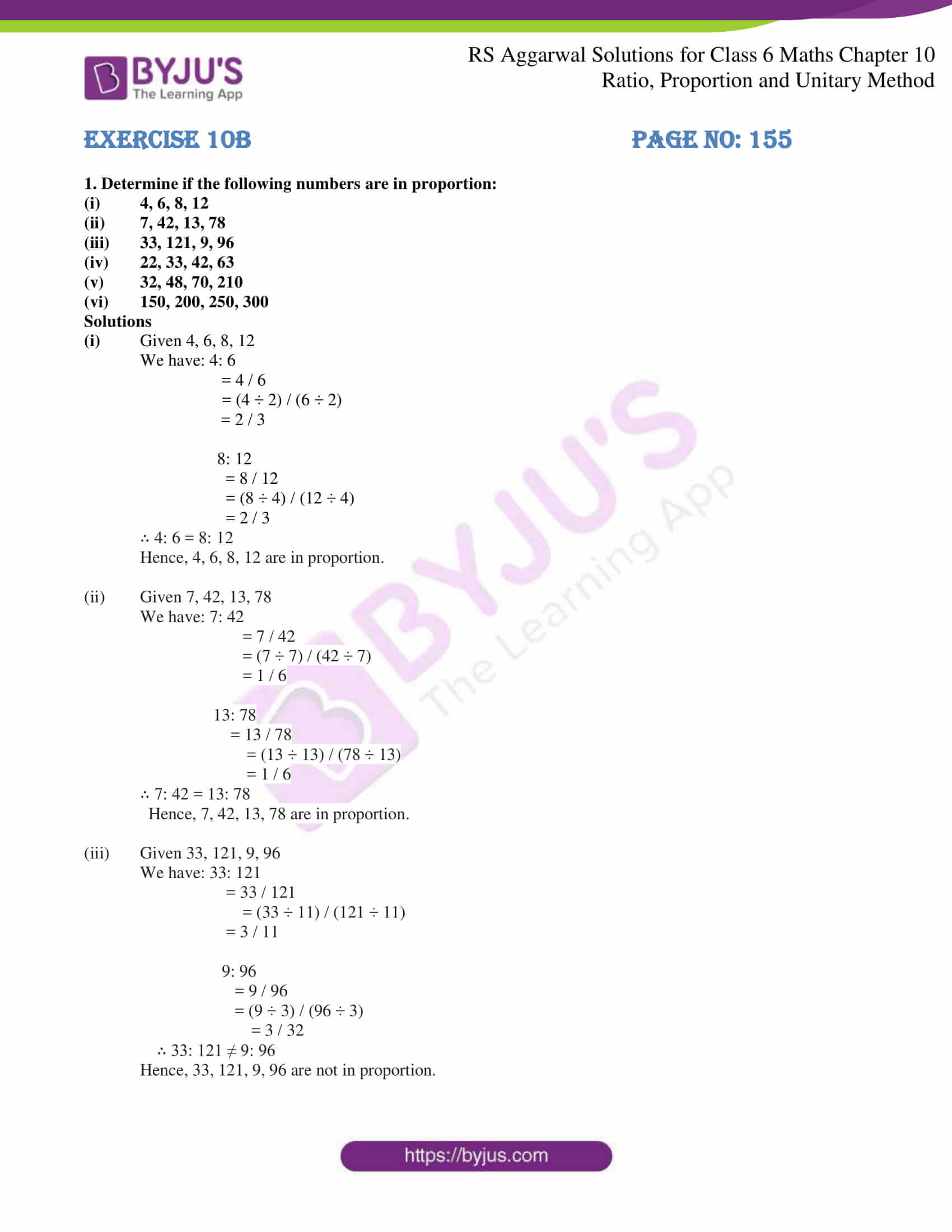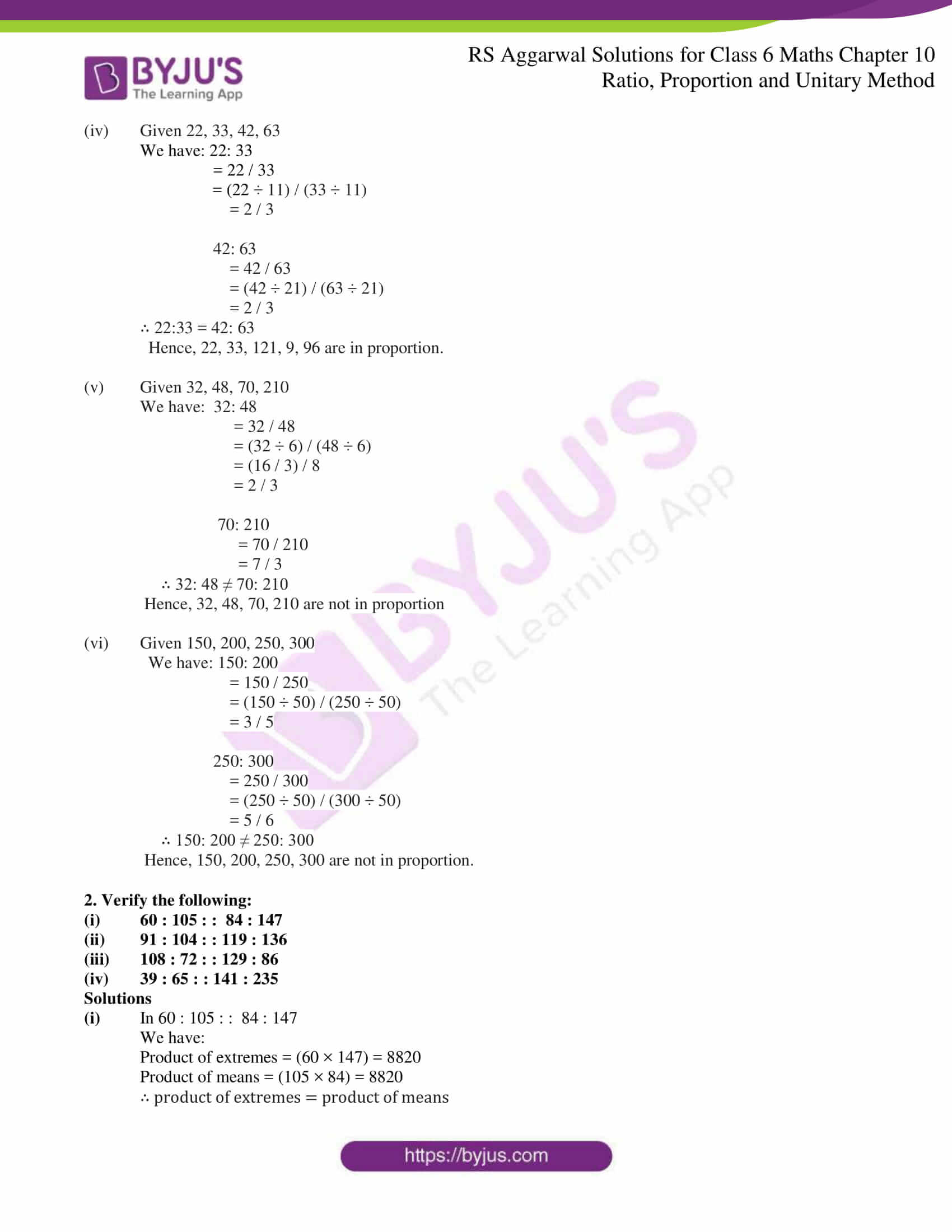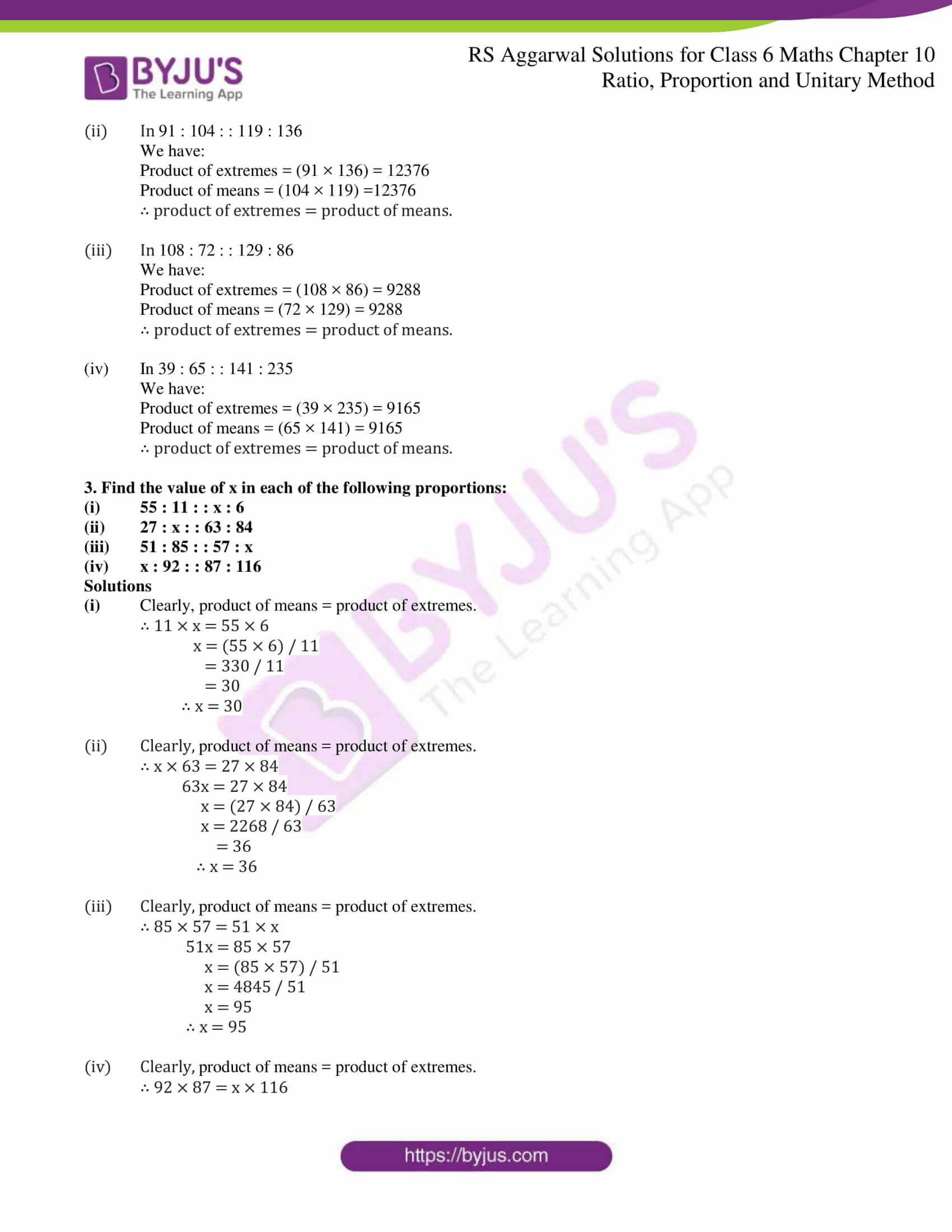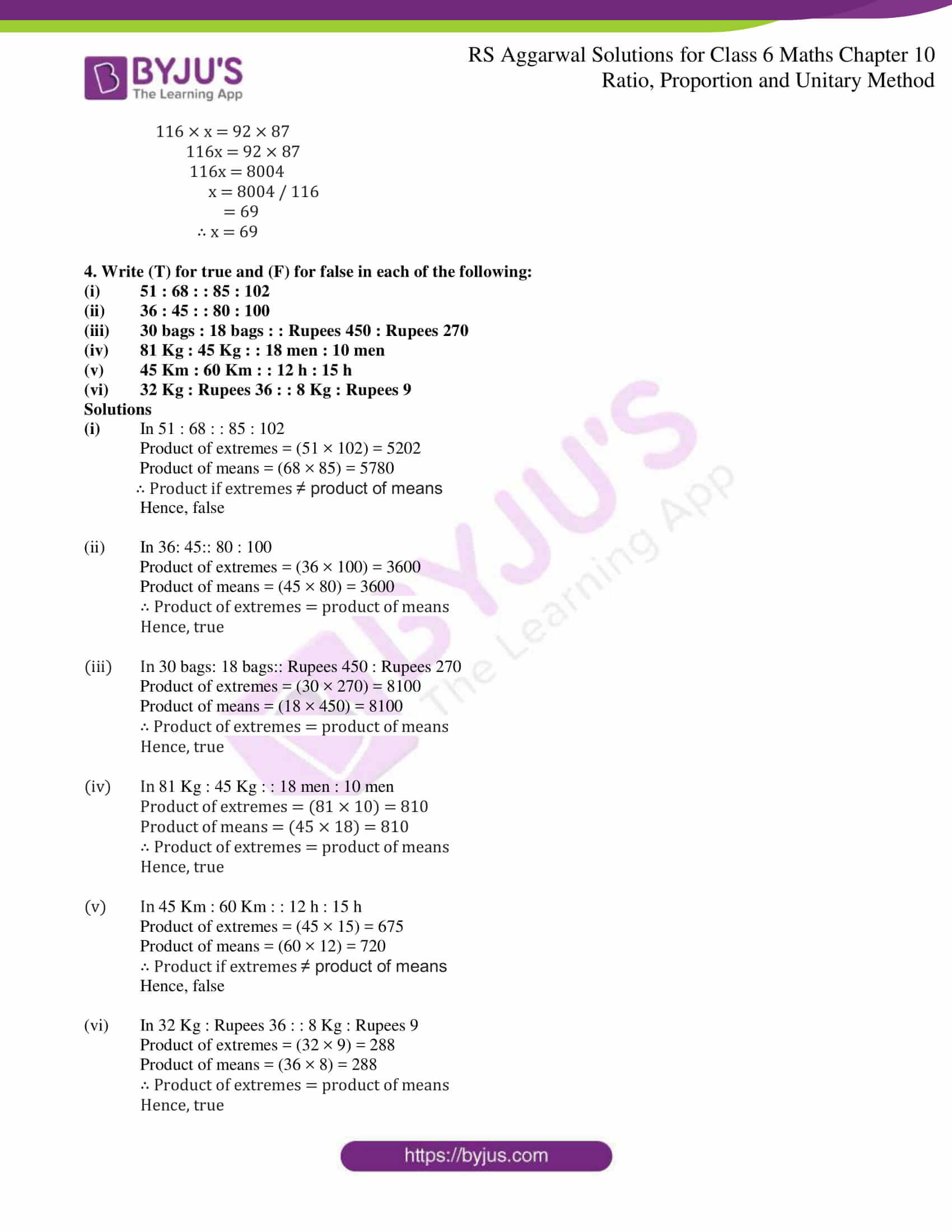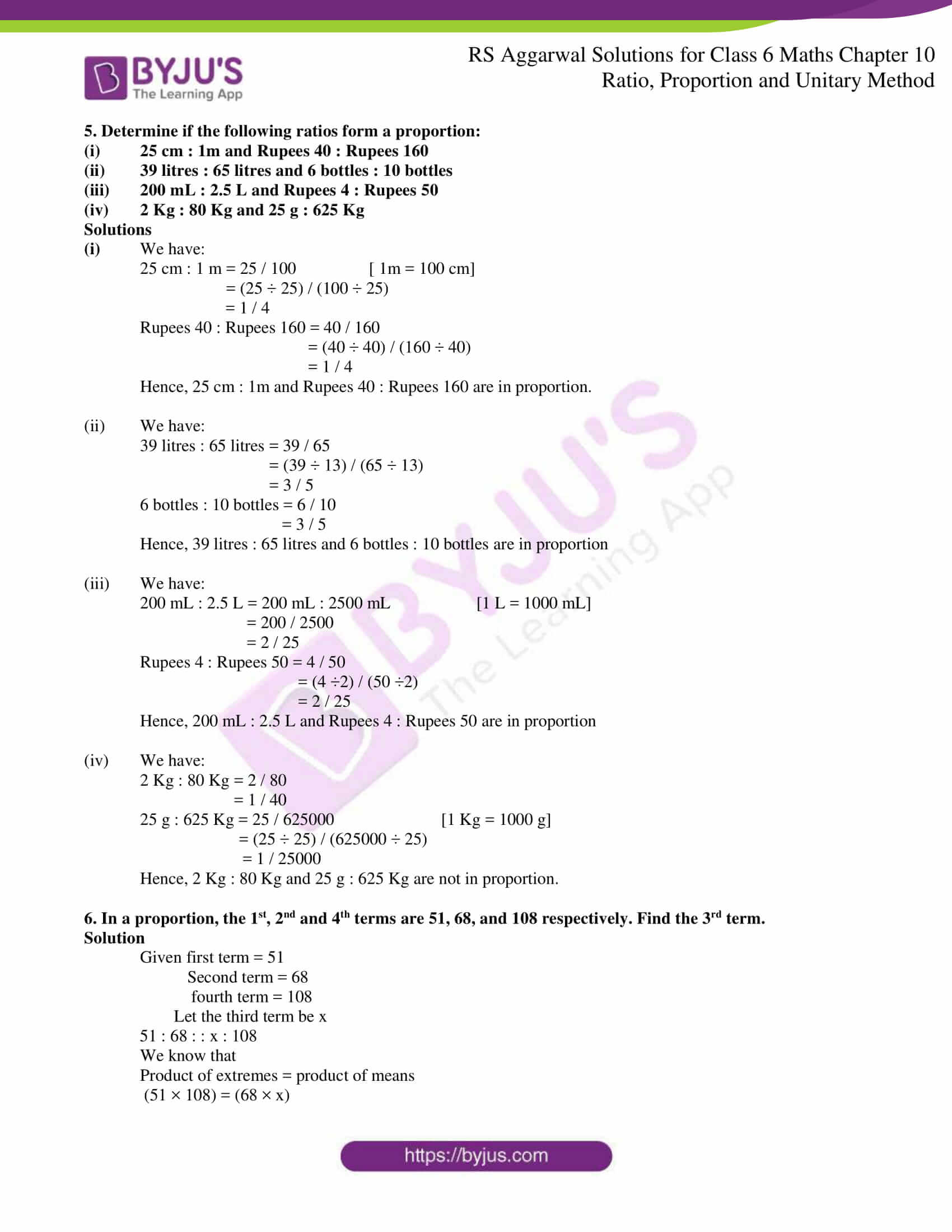### Access answers to Maths RS Aggarwal Solutions for Class 6 Chapter 10 Ratio, Proportion and Unitary Method Exercise 10B

1. Determine if the following numbers are in proportion:

(i) 4, 6, 8, 12

(ii) 7, 42, 13, 78

(iii) 33, 121, 9, 96

(iv) 22, 33, 42, 63

(v) 32, 48, 70, 210

(vi) 150, 200, 250, 300

Solutions

(i) Given 4, 6, 8, 12

We have: 4: 6

= 4 / 6

= (4 ÷ 2) / (6 ÷ 2)

= 2 / 3

8: 12

= 8 / 12

= (8 ÷ 4) / (12 ÷ 4)

= 2 / 3

∴ 4: 6 = 8: 12

Hence, 4, 6, 8, 12 are in proportion.

(ii) Given 7, 42, 13, 78

We have: 7: 42

= 7 / 42

= (7 ÷ 7) / (42 ÷ 7)

= 1 / 6

13: 78

= 13 / 78

= (13 ÷ 13) / (78 ÷ 13)

= 1 / 6

∴ 7: 42 = 13: 78

Hence, 7, 42, 13, 78 are in proportion.

(iii) Given 33, 121, 9, 96

We have: 33: 121

= 33 / 121

= (33 ÷ 11) / (121 ÷ 11)

= 3 / 11

9: 96

= 9 / 96

= (9 ÷ 3) / (96 ÷ 3)

= 3 / 32

∴ 33: 121 ≠ 9: 96

Hence, 33, 121, 9, 96 are not in proportion.

(iv) Given 22, 33, 42, 63

We have: 22: 33

= 22 / 33

= (22 ÷ 11) / (33 ÷ 11)

= 2 / 3

42: 63

= 42 / 63

= (42 ÷ 21) / (63 ÷ 21)

= 2 / 3

∴ 22:33 = 42: 63

Hence, 22, 33, 121, 9, 96 are in proportion.

(v) Given 32, 48, 70, 210

We have: 32: 48

= 32 / 48

= (32 ÷ 6) / (48 ÷ 6)

= (16 / 3) / 8

= 2 / 3

70: 210

= 70 / 210

= 7 / 3

∴ 32: 48 ≠ 70: 210

Hence, 32, 48, 70, 210 are not in proportion

(vi) Given 150, 200, 250, 300

We have: 150: 200

= 150 / 250

= (150 ÷ 50) / (250 ÷ 50)

= 3 / 5

250: 300

= 250 / 300

= (250 ÷ 50) / (300 ÷ 50)

= 5 / 6

∴ 150: 200 ≠ 250: 300

Hence, 150, 200, 250, 300 are not in proportion.

2. Verify the following:

(i) 60 : 105 : : 84 : 147

(ii) 91 : 104 : : 119 : 136

(iii) 108 : 72 : : 129 : 86

(iv) 39 : 65 : : 141 : 235

Solutions

(i) In 60 : 105 : : 84 : 147

We have:

Product of extremes = (60 × 147) = 8820

Product of means = (105 × 84) = 8820

∴ product of extremes = product of means

(ii) In 91 : 104 : : 119 : 136

We have:

Product of extremes = (91 × 136) = 12376

Product of means = (104 × 119) =12376

∴ product of extremes = product of means.

(iii) In 108 : 72 : : 129 : 86

We have:

Product of extremes = (108 × 86) = 9288

Product of means = (72 × 129) = 9288

∴ product of extremes = product of means.

(iv) In 39 : 65 : : 141 : 235

We have:

Product of extremes = (39 × 235) = 9165

Product of means = (65 × 141) = 9165

∴ product of extremes = product of means.

3. Find the value of x in each of the following proportions:

(i) 55 : 11 : : x : 6

(ii) 27 : x : : 63 : 84

(iii) 51 : 85 : : 57 : x

(iv) x : 92 : : 87 : 116

Solutions

(i) Clearly, product of means = product of extremes.

∴ 11 × x = 55 × 6

x = (55 × 6) / 11

= 330 / 11

= 30

∴ x = 30

(ii) Clearly, product of means = product of extremes.

∴ x × 63 = 27 × 84

63x = 27 × 84

x = (27 × 84) / 63

x = 2268 / 63

= 36

∴ x = 36

(iii) Clearly, product of means = product of extremes.

∴ 85 × 57 = 51 × x

51x = 85 × 57

x = (85 × 57) / 51

x = 4845 / 51

x = 95

∴ x = 95

(iv) Clearly, product of means = product of extremes.

∴ 92 × 87 = x × 116

116 × x = 92 × 87

116x = 92 × 87

116x = 8004

x = 8004 / 116

= 69

∴ x = 69

4. Write (T) for true and (F) for false in each of the following:

(i) 51 : 68 : : 85 : 102

(ii) 36 : 45 : : 80 : 100

(iii) 30 bags : 18 bags : : Rupees 450 : Rupees 270

(iv) 81 Kg : 45 Kg : : 18 men : 10 men

(v) 45 Km : 60 Km : : 12 h : 15 h

(vi) 32 Kg : Rupees 36 : : 8 Kg : Rupees 9

Solutions

(i) In 51 : 68 : : 85 : 102

Product of extremes = (51 × 102) = 5202

Product of means = (68 × 85) = 5780

∴ Product if extremes ≠ product of means

Hence, false

(ii) In 36: 45:: 80 : 100

Product of extremes = (36 × 100) = 3600

Product of means = (45 × 80) = 3600

∴ Product of extremes = product of means

Hence, true

(iii) In 30 bags: 18 bags:: Rupees 450 : Rupees 270

Product of extremes = (30 × 270) = 8100

Product of means = (18 × 450) = 8100

∴ Product of extremes = product of means

Hence, true

(iv) In 81 Kg : 45 Kg : : 18 men : 10 men

Product of extremes = (81 × 10) = 810

Product of means = (45 × 18) = 810

∴ Product of extremes = product of means

Hence, true

(v) In 45 Km : 60 Km : : 12 h : 15 h

Product of extremes = (45 × 15) = 675

Product of means = (60 × 12) = 720

∴ Product if extremes ≠ product of means

Hence, false

(vi) In 32 Kg : Rupees 36 : : 8 Kg : Rupees 9

Product of extremes = (32 × 9) = 288

Product of means = (36 × 8) = 288

∴ Product of extremes = product of means

Hence, true

5. Determine if the following ratios form a proportion:

(i) 25 cm : 1m and Rupees 40 : Rupees 160

(ii) 39 litres : 65 litres and 6 bottles : 10 bottles

(iii) 200 mL : 2.5 L and Rupees 4 : Rupees 50

(iv) 2 Kg : 80 Kg and 25 g : 625 Kg

Solutions

(i) We have:

25 cm : 1 m = 25 / 100 [ 1m = 100 cm]

= (25 ÷ 25) / (100 ÷ 25)

= 1 / 4

Rupees 40 : Rupees 160 = 40 / 160

= (40 ÷ 40) / (160 ÷ 40)

= 1 / 4

Hence, 25 cm : 1m and Rupees 40 : Rupees 160 are in proportion.

(ii) We have:

39 litres : 65 litres = 39 / 65

= (39 ÷ 13) / (65 ÷ 13)

= 3 / 5

6 bottles : 10 bottles = 6 / 10

= 3 / 5

Hence, 39 litres : 65 litres and 6 bottles : 10 bottles are in proportion

(iii) We have:

200 mL : 2.5 L = 200 mL : 2500 mL [1 L = 1000 mL]

= 200 / 2500

= 2 / 25

Rupees 4 : Rupees 50 = 4 / 50

= (4 ÷2) / (50 ÷2)

= 2 / 25

Hence, 200 mL : 2.5 L and Rupees 4 : Rupees 50 are in proportion

(iv) We have:

2 Kg : 80 Kg = 2 / 80

= 1 / 40

25 g : 625 Kg = 25 / 625000 [1 Kg = 1000 g]

= (25 ÷ 25) / (625000 ÷ 25)

= 1 / 25000

Hence, 2 Kg : 80 Kg and 25 g : 625 Kg are not in proportion.

6. In a proportion, the 1st, 2nd and 4th terms are 51, 68, and 108 respectively. Find the 3rd term.

Solution

Given first term = 51

Second term = 68

fourth term = 108

Let the third term be x

51 : 68 : : x : 108

We know that

Product of extremes = product of means

(51 × 108) = (68 × x)

(68 × x) = (51 × 108)

68x = 5508

x = 5508 / 68